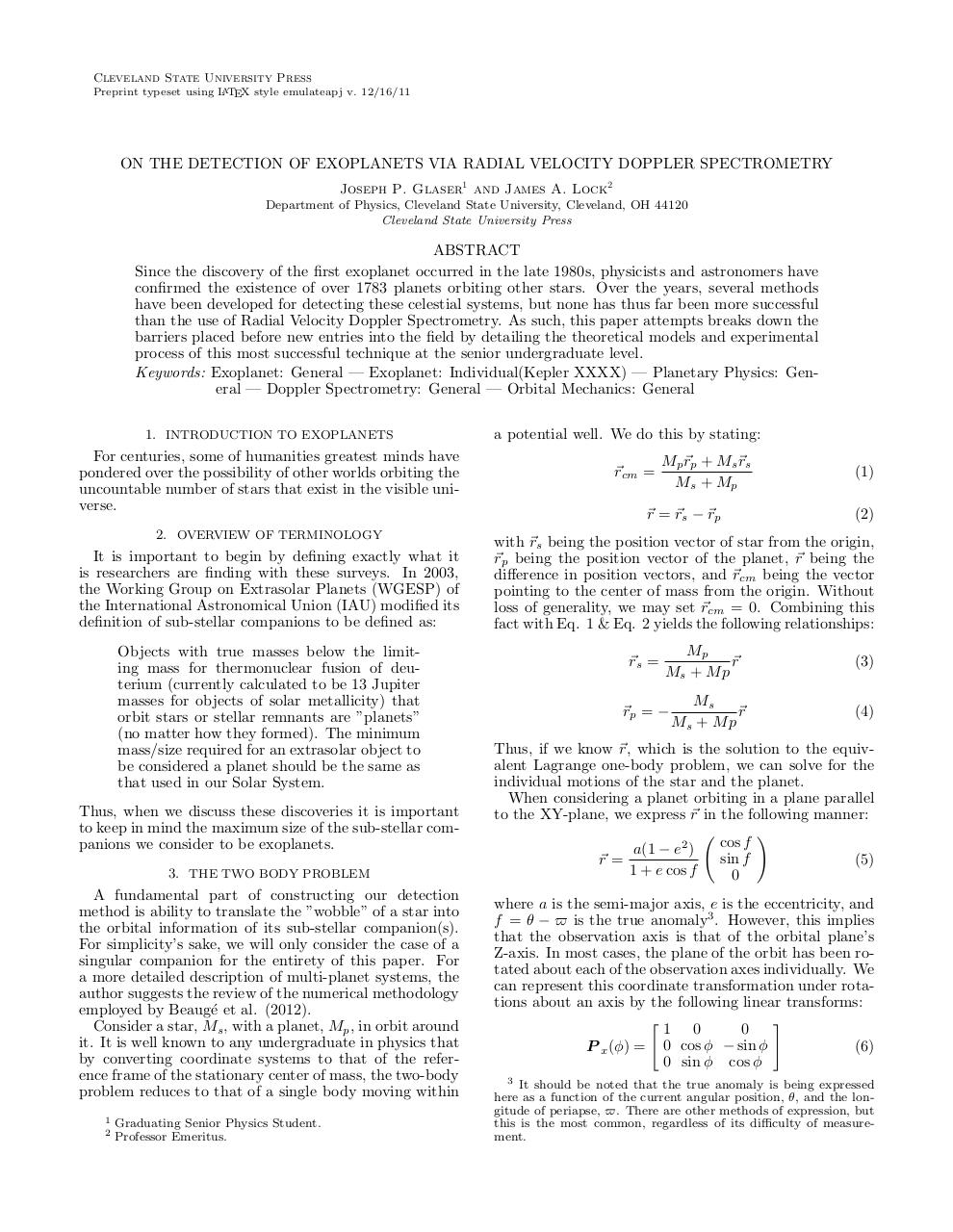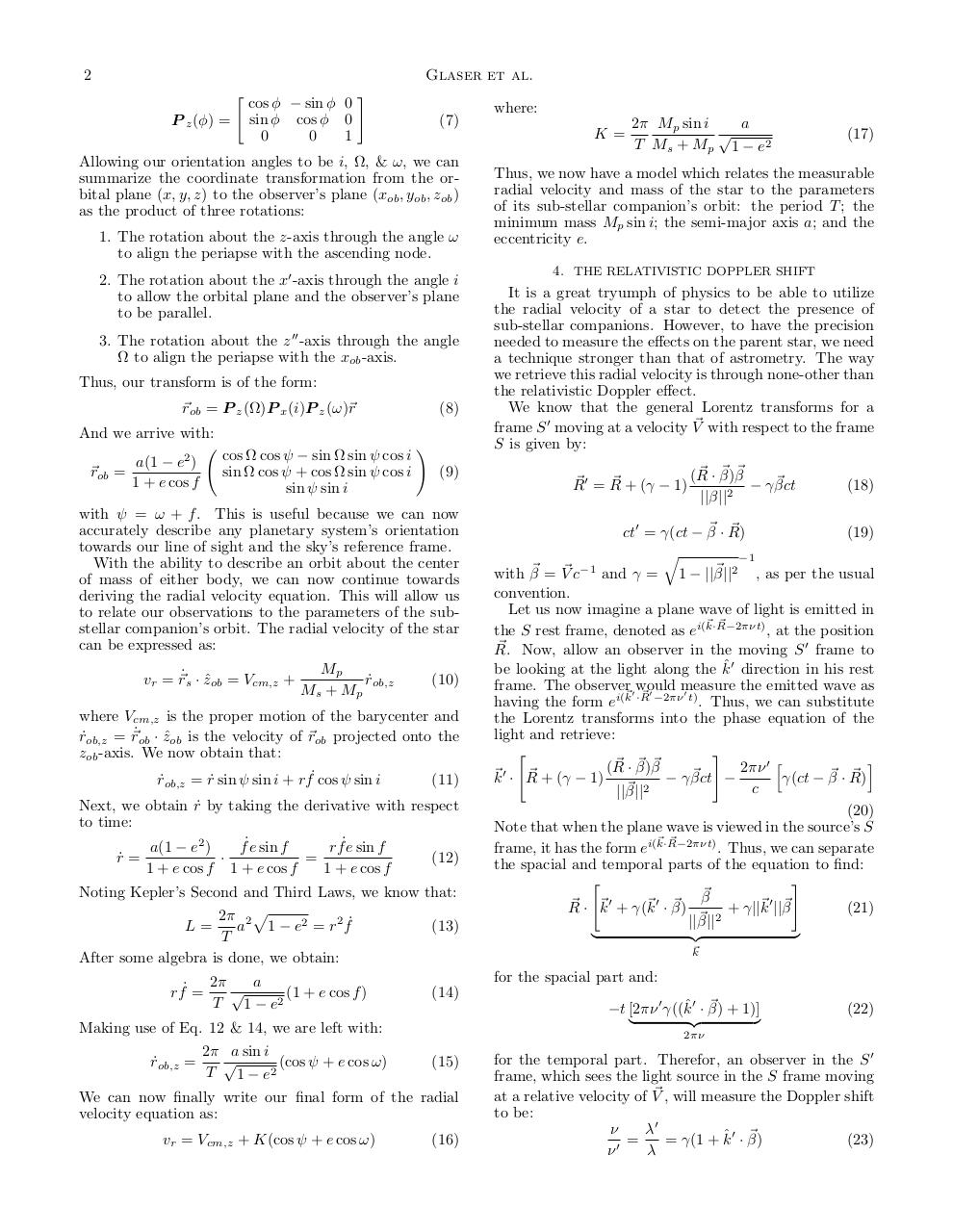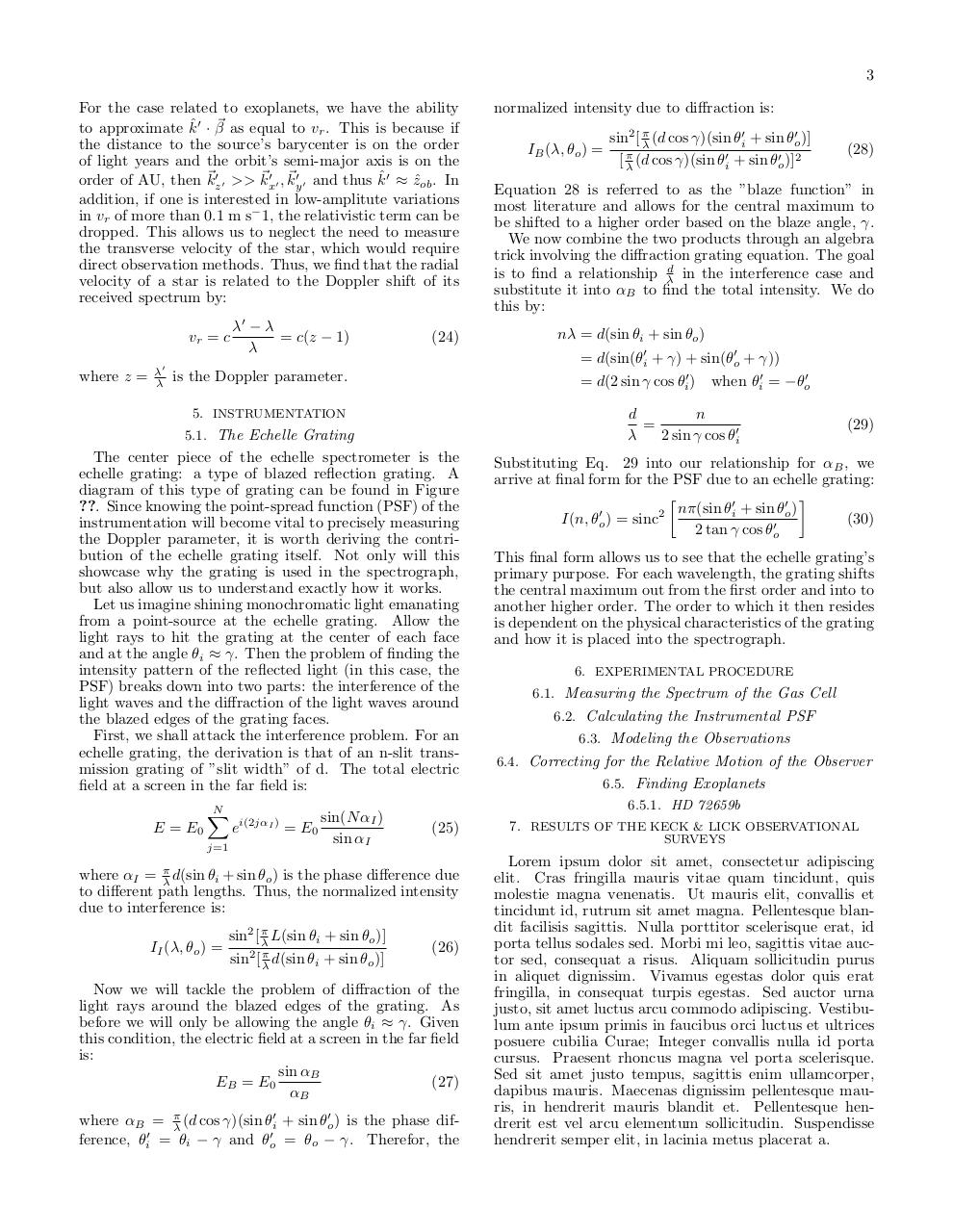# Exoplanet Paper 4514 .pdf

### File information

Original filename: Exoplanet_Paper_4514.pdf

This PDF 1.5 document has been generated by TeX / pdfTeX-1.40.14, and has been sent on pdf-archive.com on 06/05/2014 at 05:14, from IP address 108.90.x.x. The current document download page has been viewed 583 times.
File size: 246 KB (5 pages).
Privacy: public file

Exoplanet_Paper_4514.pdf (PDF, 246 KB)

### Document preview

Cleveland State University Press
Preprint typeset using LATEX style emulateapj v. 12/16/11

ON THE DETECTION OF EXOPLANETS VIA RADIAL VELOCITY DOPPLER SPECTROMETRY
Joseph P. Glaser1 and James A. Lock2
Department of Physics, Cleveland State University, Cleveland, OH 44120
Cleveland State University Press

ABSTRACT
Since the discovery of the first exoplanet occurred in the late 1980s, physicists and astronomers have
confirmed the existence of over 1783 planets orbiting other stars. Over the years, several methods
have been developed for detecting these celestial systems, but none has thus far been more successful
than the use of Radial Velocity Doppler Spectrometry. As such, this paper attempts breaks down the
barriers placed before new entries into the field by detailing the theoretical models and experimental
process of this most successful technique at the senior undergraduate level.
Keywords: Exoplanet: General — Exoplanet: Individual(Kepler XXXX) — Planetary Physics: General — Doppler Spectrometry: General — Orbital Mechanics: General
1. INTRODUCTION TO EXOPLANETS

For centuries, some of humanities greatest minds have
pondered over the possibility of other worlds orbiting the
uncountable number of stars that exist in the visible universe.
2. OVERVIEW OF TERMINOLOGY

It is important to begin by defining exactly what it
is researchers are finding with these surveys. In 2003,
the Working Group on Extrasolar Planets (WGESP) of
the International Astronomical Union (IAU) modified its
definition of sub-stellar companions to be defined as:
Objects with true masses below the limiting mass for thermonuclear fusion of deuterium (currently calculated to be 13 Jupiter
masses for objects of solar metallicity) that
orbit stars or stellar remnants are ”planets”
(no matter how they formed). The minimum
mass/size required for an extrasolar object to
be considered a planet should be the same as
that used in our Solar System.
Thus, when we discuss these discoveries it is important
to keep in mind the maximum size of the sub-stellar companions we consider to be exoplanets.
3. THE TWO BODY PROBLEM

A fundamental part of constructing our detection
method is ability to translate the ”wobble” of a star into
the orbital information of its sub-stellar companion(s).
For simplicity’s sake, we will only consider the case of a
singular companion for the entirety of this paper. For
a more detailed description of multi-planet systems, the
author suggests the review of the numerical methodology
employed by Beaug´e et al. (2012).
Consider a star, Ms , with a planet, Mp , in orbit around
it. It is well known to any undergraduate in physics that
by converting coordinate systems to that of the reference frame of the stationary center of mass, the two-body
problem reduces to that of a single body moving within
1
2

Professor Emeritus.

a potential well. We do this by stating:
~rcm =

Mp~rp + Ms~rs
Ms + Mp

~r = ~rs − ~rp

(1)
(2)

with ~rs being the position vector of star from the origin,
~rp being the position vector of the planet, ~r being the
difference in position vectors, and ~rcm being the vector
pointing to the center of mass from the origin. Without
loss of generality, we may set ~rcm = 0. Combining this
fact with Eq. 1 &amp; Eq. 2 yields the following relationships:
~rs =

Mp
~r
Ms + M p

~rp = −

Ms
~r
Ms + M p

(3)
(4)

Thus, if we know ~r, which is the solution to the equivalent Lagrange one-body problem, we can solve for the
individual motions of the star and the planet.
When considering a planet orbiting in a plane parallel
to the XY-plane, we express ~r in the following manner:
!
cos f
a(1 − e2 )
sin f
(5)
~r =
1 + e cos f
0
where a is the semi-major axis, e is the eccentricity, and
f = θ − \$ is the true anomaly3 . However, this implies
that the observation axis is that of the orbital plane’s
Z-axis. In most cases, the plane of the orbit has been rotated about each of the observation axes individually. We
can represent this coordinate transformation under rotations about an axis by the following linear transforms:
"
#
1 0
0
P x (φ) = 0 cos φ − sin φ
(6)
0 sin φ cos φ
3 It should be noted that the true anomaly is being expressed
here as a function of the current angular position, θ, and the longitude of periapse, \$. There are other methods of expression, but
this is the most common, regardless of its difficulty of measurement.

2

Glaser et al.
"
P z (φ) =

cos φ − sin φ 0
sin φ cos φ 0
0
0
1

#
(7)

K=

Allowing our orientation angles to be i, Ω, &amp; ω, we can
summarize the coordinate transformation from the orbital plane (x, y, z) to the observer’s plane (xob , yob , zob )
as the product of three rotations:
1. The rotation about the z-axis through the angle ω
to align the periapse with the ascending node.
2. The rotation about the x0 -axis through the angle i
to allow the orbital plane and the observer’s plane
to be parallel.
3. The rotation about the z 00 -axis through the angle
Ω to align the periapse with the xob -axis.
Thus, our transform is of the form:
~rob = P z (Ω)P x (i)P z (ω)~r

(8)

And we arrive with:
~rob

a(1 − e2 )
=
1 + e cos f

cos Ω cos ψ − sin Ω sin ψ cos i
sin Ω cos ψ + cos Ω sin ψ cos i
sin ψ sin i

!
(9)

with ψ = ω + f . This is useful because we can now
accurately describe any planetary system’s orientation
towards our line of sight and the sky’s reference frame.
With the ability to describe an orbit about the center
of mass of either body, we can now continue towards
deriving the radial velocity equation. This will allow us
to relate our observations to the parameters of the substellar companion’s orbit. The radial velocity of the star
can be expressed as:
vr = ~r˙s · zˆob = Vcm,z +

Mp
r˙ob,z
Ms + Mp

(10)

where Vcm,z is the proper motion of the barycenter and
r˙ob,z = ~r˙ob · zˆob is the velocity of ~rob projected onto the
zob -axis. We now obtain that:
r˙ob,z = r˙ sin ψ sin i + rf˙ cos ψ sin i
(11)
Next, we obtain r˙ by taking the derivative with respect
to time:
a(1 − e2 )
f˙e sin f
rf˙e sin f
r˙ =
·
=
(12)
1 + e cos f 1 + e cos f
1 + e cos f
Noting Kepler’s Second and Third Laws, we know that:
2π 2 p
L=
a 1 − e2 = r2 f˙
(13)
T
After some algebra is done, we obtain:

a

rf˙ =
(1 + e cos f )
T 1 − e2

r˙ob,z

(17)

Thus, we now have a model which relates the measurable
radial velocity and mass of the star to the parameters
of its sub-stellar companion’s orbit: the period T ; the
minimum mass Mp sin i; the semi-major axis a; and the
eccentricity e.
4. THE RELATIVISTIC DOPPLER SHIFT

It is a great tryumph of physics to be able to utilize
the radial velocity of a star to detect the presence of
sub-stellar companions. However, to have the precision
needed to measure the effects on the parent star, we need
a technique stronger than that of astrometry. The way
we retrieve this radial velocity is through none-other than
the relativistic Doppler effect.
We know that the general Lorentz transforms for a
~ with respect to the frame
frame S 0 moving at a velocity V
S is given by:
~ ~ ~
~
~0 = R
~ + (γ − 1) (R · β)β − γ βct
R
||β||2

(18)

~
ct0 = γ(ct − β~ · R)
(19)
q
−1
~ 2 , as per the usual
and γ = 1 − ||β||

~ c−1
with β~ = V
convention.
Let us now imagine a plane wave of light is emitted in
~ ~
the S rest frame, denoted as ei(k·R−2πνt) , at the position
~ Now, allow an observer in the moving S 0 frame to
R.
be looking at the light along the kˆ0 direction in his rest
frame. The observer 0would
measure
the emitted wave as
0
~ ~0
having the form ei(k ·R −2πν t) . Thus, we can substitute
the Lorentz transforms into the phase equation of the
light and retrieve:
"
#
i
~ β~
~ · β)
(
R
2πν 0 h
0
~k · R
~
~ + (γ − 1)
~
− γ βct

γ(ct − β~ · R)
~ 2
c
||β||
(20)
Note that when the plane wave is viewed in the source’s S
~ ~
frame, it has the form ei(k·R−2πνt) . Thus, we can separate
the spacial and temporal parts of the equation to find:
"
#
~
β
0
0
0
~
~
~
~
~
~ · k + γ(k · β)
R
+ γ||k ||β
(21)
~ 2
||β||
|
{z
}
~
k

~ + 1)]
−t [2πν 0 γ((kˆ0 · β)
|
{z
}

(22)

2πν

(15)

We can now finally write our final form of the radial
velocity equation as:
vr = Vcm,z + K(cos ψ + e cos ω)

a
2π Mp sin i

T Ms + Mp 1 − e 2

for the spacial part and:
(14)

Making use of Eq. 12 &amp; 14, we are left with:
2π a sin i

=
(cos ψ + e cos ω)
T 1 − e2

where:

(16)

for the temporal part. Therefor, an observer in the S 0
frame, which sees the light source in the S frame moving
~ , will measure the Doppler shift
at a relative velocity of V
to be:
ν
λ0
~
=
= γ(1 + kˆ0 · β)
(23)
0
ν
λ

3
For the case related to exoplanets, we have the ability
to approximate kˆ0 · β~ as equal to vr . This is because if
the distance to the source’s barycenter is on the order
of light years and the orbit’s semi-major axis is on the
order of AU, then ~kz0 0 &gt;&gt; ~kx0 0 , ~ky0 0 and thus kˆ0 ≈ zˆob . In
addition, if one is interested in low-amplitute variations
in vr of more than 0.1 m s− 1, the relativistic term can be
dropped. This allows us to neglect the need to measure
the transverse velocity of the star, which would require
direct observation methods. Thus, we find that the radial
velocity of a star is related to the Doppler shift of its
vr = c
where z =

λ0
λ

λ0 − λ
= c(z − 1)
λ

(24)

5. INSTRUMENTATION

j=1

sin(N αI )
sin αI

(25)

where αI = πλ d(sin θi + sin θo ) is the phase difference due
to different path lengths. Thus, the normalized intensity
due to interference is:
II (λ, θo ) =

sin2 [ πλ L(sin θi + sin θo )]
sin2 [ πλ d(sin θi + sin θo )]

(28)

Equation 28 is referred to as the ”blaze function” in
most literature and allows for the central maximum to
be shifted to a higher order based on the blaze angle, γ.
We now combine the two products through an algebra
trick involving the diffraction grating equation. The goal
is to find a relationship λd in the interference case and
substitute it into αB to find the total intensity. We do
this by:
nλ = d(sin θi + sin θo )
when θi0 = −θo0

d
n
=
λ
2 sin γ cos θi0

The center piece of the echelle spectrometer is the
echelle grating: a type of blazed reflection grating. A
diagram of this type of grating can be found in Figure
??. Since knowing the point-spread function (PSF) of the
instrumentation will become vital to precisely measuring
the Doppler parameter, it is worth deriving the contribution of the echelle grating itself. Not only will this
showcase why the grating is used in the spectrograph,
but also allow us to understand exactly how it works.
Let us imagine shining monochromatic light emanating
from a point-source at the echelle grating. Allow the
light rays to hit the grating at the center of each face
and at the angle θi ≈ γ. Then the problem of finding the
intensity pattern of the reflected light (in this case, the
PSF) breaks down into two parts: the interference of the
light waves and the diffraction of the light waves around
the blazed edges of the grating faces.
First, we shall attack the interference problem. For an
echelle grating, the derivation is that of an n-slit transmission grating of ”slit width” of d. The total electric
field at a screen in the far field is:
ei(2jαI ) = E0

sin2 [ πλ (d cos γ)(sin θi0 + sin θo0 )]
[ πλ (d cos γ)(sin θi0 + sin θo0 )]2

= d(2 sin γ cos θi0 )

5.1. The Echelle Grating

N
X

IB (λ, θo ) =

= d(sin(θi0 + γ) + sin(θo0 + γ))

is the Doppler parameter.

E = E0

normalized intensity due to diffraction is:

(26)

Now we will tackle the problem of diffraction of the
light rays around the blazed edges of the grating. As
before we will only be allowing the angle θi ≈ γ. Given
this condition, the electric field at a screen in the far field
is:
sin αB
EB = E0
(27)
αB
where αB = πλ (d cos γ)(sin θi0 + sin θo0 ) is the phase difference, θi0 = θi − γ and θo0 = θo − γ. Therefor, the

(29)

Substituting Eq. 29 into our relationship for αB , we
arrive at final form for the PSF due to an echelle grating:


0
0
2 nπ(sin θi + sin θo )
0
(30)
I(n, θo ) = sinc
2 tan γ cos θo0
This final form allows us to see that the echelle grating’s
primary purpose. For each wavelength, the grating shifts
the central maximum out from the first order and into to
another higher order. The order to which it then resides
is dependent on the physical characteristics of the grating
and how it is placed into the spectrograph.
6. EXPERIMENTAL PROCEDURE
6.1. Measuring the Spectrum of the Gas Cell
6.2. Calculating the Instrumental PSF
6.3. Modeling the Observations
6.4. Correcting for the Relative Motion of the Observer
6.5. Finding Exoplanets
6.5.1. HD 72659b
7. RESULTS OF THE KECK &amp; LICK OBSERVATIONAL
SURVEYS

Lorem ipsum dolor sit amet, consectetur adipiscing
elit. Cras fringilla mauris vitae quam tincidunt, quis
molestie magna venenatis. Ut mauris elit, convallis et
tincidunt id, rutrum sit amet magna. Pellentesque blandit facilisis sagittis. Nulla porttitor scelerisque erat, id
porta tellus sodales sed. Morbi mi leo, sagittis vitae auctor sed, consequat a risus. Aliquam sollicitudin purus
in aliquet dignissim. Vivamus egestas dolor quis erat
fringilla, in consequat turpis egestas. Sed auctor urna
justo, sit amet luctus arcu commodo adipiscing. Vestibulum ante ipsum primis in faucibus orci luctus et ultrices
posuere cubilia Curae; Integer convallis nulla id porta
cursus. Praesent rhoncus magna vel porta scelerisque.
Sed sit amet justo tempus, sagittis enim ullamcorper,
dapibus mauris. Maecenas dignissim pellentesque mauris, in hendrerit mauris blandit et. Pellentesque hendrerit est vel arcu elementum sollicitudin. Suspendisse
hendrerit semper elit, in lacinia metus placerat a.

4

Glaser et al.
8. CONCLUDING THOUGHTS

We are grateful to: the Department of Physics at
Cleveland State University for facilitating the course
which allowed this study to be done; the Honors Scholarship which funded J. Glaser during his studies; and J.
Lock for his guidance and support throughout the project
and years previous to its completion.
APPENDIX
APPENDIX MATERIAL

REFERENCES
Auri`
ere, M. 1982, A&amp;A, 109, 301

5
Figure 1. Derived spectra for 3C138 (see ?). Plots for all sources are available in the electronic edition of The Astrophysical Journal.